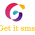# What is the factorial of 100? (hundred)

The number 100! (pronounced “one hundred factorial”) is the number you get when you multiply all the whole numbers from 1 to 100.
That is, 100! = 1×2×3×…×99×100.
When this number is calculated and written out in full, how many zeros are on the end?

# What is the factorial of 100? (hundred)

100 x 99 x 98 x 97 x 96 x ... = 9.3326215443944E+157

## What is factorial?

### Definition of factorial

The factorial is a quantity defined for any integer n greater than or equal to 0.

The factorial is the product of all integers less than or equal to n but greater than or equal to 1. The factorial value of 0 is by definition equal to 1. For negative integers, factorials are not defined. The factorial can be seen as the result of multiplying a sequence of descending natural numbers (such as 3 × 2 × 1).

The factorial symbol is the exclamation mark !.

### The factorial formula

If n is a natural number greater than or equal to 1, then

n! = n x (n - 1) x (n - 2) x (n - 3) ... 3 x 2 x 1

If n = 0, then n! = 1, by convention.

Example: 6! = 6 x 5 x 4 x 3 x 2 x 1 = 720

Factorials are used in math quite a lot when calculating the number of possible combinations or permutations of something. If you think about shuffling a deck of 52 cards, you can use factorials to calculate how many possible orders there are.

Factorial Tables Chart 1! to 100!
1! = 1
2! = 2
3! = 6
4! = 24
5! = 120
6! = 720
7! = 5040
8! = 40320
9! = 362880
10! = 3628800
11! = 39916800
12! = 479001600
13! = 6227020800
14! = 87178291200
15! = 1307674368000
16! = 20922789888000
17! = 355687428096000
18! = 6402373705728000
19! = 121645100408832000
20! = 2432902008176640000
21! = 51090942171709440000
22! = 1124000727777607680000
23! = 25852016738884976640000
24! = 620448401733239439360000
25! = 15511210043330985984000000
26! = 403291461126605635584000000
27! = 10888869450418352160768000000
28! = 304888344611713860501504000000
29! = 8841761993739701954543616000000
30! = 265252859812191058636308480000000
31! = 8222838654177922817725562880000000
32! = 263130836933693530167218012160000000
33! = 8683317618811886495518194401280000000
34! = 295232799039604140847618609643520000000
35! = 10333147966386144929666651337523200000000
36! = 371993326789901217467999448150835200000000
37! = 13763753091226345046315979581580902400000000
38! = 523022617466601111760007224100074291200000000
39! = 20397882081197443358640281739902897356800000000
40! = 815915283247897734345611269596115894272000000000
41! = 33452526613163807108170062053440751665152000000000
42! = 1405006117752879898543142606244511569936384000000000
43! = 60415263063373835637355132068513997507264512000000000
44! = 2658271574788448768043625811014615890319638528000000000
45! = 119622220865480194561963161495657715064383733760000000000
46! = 5502622159812088949850305428800254892961651752960000000000
47! = 258623241511168180642964355153611979969197632389120000000000
48! = 12413915592536072670862289047373375038521486354677760000000000
49! = 608281864034267560872252163321295376887552831379210240000000000
50! = 30414093201713378043612608166064768844377641568960512000000000000
51! = 1551118753287382280224243016469303211063259720016986112000000000000
52! = 80658175170943878571660636856403766975289505440883277824000000000000
53! = 4274883284060025564298013753389399649690343788366813724672000000000000
54! = 230843697339241380472092742683027581083278564571807941132288000000000000
55! = 12696403353658275925965100847566516959580321051449436762275840000000000000
56! = 710998587804863451854045647463724949736497978881168458687447040000000000000
57! = 40526919504877216755680601905432322134980384796226602145184481280000000000000
58! = 2350561331282878571829474910515074683828862318181142924420699914240000000000000
59! = 138683118545689835737939019720389406345902876772687432540821294940160000000000000
60! = 8320987112741390144276341183223364380754172606361245952449277696409600000000000000
61! = 507580213877224798800856812176625227226004528988036003099405939480985600000000000000
62! = 31469973260387937525653122354950764088012280797258232192163168247821107200000000000000
63! = 1982608315404440064116146708361898137544773690227268628106279599612729753600000000000000
64! = 126886932185884164103433389335161480802865516174545192198801894375214704230400000000000000
65! = 8247650592082470666723170306785496252186258551345437492922123134388955774976000000000000000
66! = 544344939077443064003729240247842752644293064388798874532860126869671081148416000000000000000
67! = 36471110918188685288249859096605464427167635314049524593701628500267962436943872000000000000000
68! = 2480035542436830599600990418569171581047399201355367672371710738018221445712183296000000000000000
69! = 171122452428141311372468338881272839092270544893520369393648040923257279754140647424000000000000000
70! = 11978571669969891796072783721689098736458938142546425857555362864628009582789845319680000000000000000
71! = 850478588567862317521167644239926010288584608120796235886430763388588680378079017697280000000000000000
72! = 61234458376886086861524070385274672740778091784697328983823014963978384987221689274204160000000000000000
73! = 4470115461512684340891257138125051110076800700282905015819080092370422104067183317016903680000000000000000
74! = 330788544151938641225953028221253782145683251820934971170611926835411235700971565459250872320000000000000000
75! = 24809140811395398091946477116594033660926243886570122837795894512655842677572867409443815424000000000000000000
76! = 1885494701666050254987932260861146558230394535379329335672487982961844043495537923117729972224000000000000000000
77! = 145183092028285869634070784086308284983740379224208358846781574688061991349156420080065207861248000000000000000000
78! = 11324281178206297831457521158732046228731749579488251990048962825668835325234200766245086213177344000000000000000000
79! = 894618213078297528685144171539831652069808216779571907213868063227837990693501860533361810841010176000000000000000000
80! = 71569457046263802294811533723186532165584657342365752577109445058227039255480148842668944867280814080000000000000000000
81! = 5797126020747367985879734231578109105412357244731625958745865049716390179693892056256184534249745940480000000000000000000
82! = 475364333701284174842138206989404946643813294067993328617160934076743994734899148613007131808479167119360000000000000000000
83! = 39455239697206586511897471180120610571436503407643446275224357528369751562996629334879591940103770870906880000000000000000000
84! = 3314240134565353266999387579130131288000666286242049487118846032383059131291716864129885722968716753156177920000000000000000000
85! = 281710411438055027694947944226061159480056634330574206405101912752560026159795933451040286452340924018275123200000000000000000000
86! = 24227095383672732381765523203441259715284870552429381750838764496720162249742450276789464634901319465571660595200000000000000000000
87! = 2107757298379527717213600518699389595229783738061356212322972511214654115727593174080683423236414793504734471782400000000000000000000
88! = 185482642257398439114796845645546284380220968949399346684421580986889562184028199319100141244804501828416633516851200000000000000000000
89! = 16507955160908461081216919262453619309839666236496541854913520707833171034378509739399912570787600662729080382999756800000000000000000000
90! = 1485715964481761497309522733620825737885569961284688766942216863704985393094065876545992131370884059645617234469978112000000000000000000000
91! = 135200152767840296255166568759495142147586866476906677791741734597153670771559994765685283954750449427751168336768008192000000000000000000000
92! = 12438414054641307255475324325873553077577991715875414356840239582938137710983519518443046123837041347353107486982656753664000000000000000000000 td>
93! = 1156772507081641574759205162306240436214753229576413535186142281213246807121467315215203289516844845303838996289387078090752000000000000000000000
94! = 108736615665674308027365285256786601004186803580182872307497374434045199869417927630229109214583415458560865651202385340530688000000000000000000000
95! = 10329978488239059262599702099394727095397746340117372869212250571234293987594703124871765375385424468563282236864226607350415360000000000000000000000
96! = 991677934870949689209571401541893801158183648651267795444376054838492222809091499987689476037000748982075094738965754305639874560000000000000000000000
97! = 96192759682482119853328425949563698712343813919172976158104477319333745612481875498805879175589072651261284189679678167647067832320000000000000000000000
98! = 9426890448883247745626185743057242473809693764078951663494238777294707070023223798882976159207729119823605850588608460429412647567360000000000000000000000
99! = 933262154439441526816992388562667004907159682643816214685929638952175999932299156089414639761565182862536979208272237582511852109168640000000000000000000000
100! = 9.33262154439441e+157

## TRICK to FIND THE FACTORIAL OF 100 (HUNDRED)

The clever bit here is thinking what numbers when multiplied together will end in a zero.
So the product of what numbers when multiplied ends in a zero:
1. When one of the things being multiplied ends in zero itself
2. A number ending in 5 multiplied by an even number

3. 25, 50 and 75 when multiplied by some of the small numbers available eg (4, 2 and 6) generate an extra zero

Below is tabulated the origin of all the zeros:

 Number Zeros Number Zeros 100 2 95 1 90 1 85 1 80 1 75 2 70 1 65 1 60 1 55 1 50 2 45 1 40 1 35 1 30 1 25 2 20 1 15 1 10 1 5 1 Total 12 Total 12

So that's it then there are 24 zeros at the end of 100!

1.2.3.